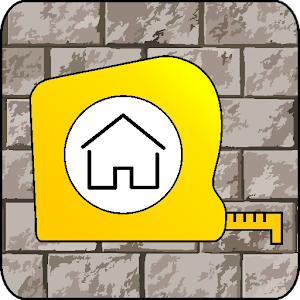CONSTRUCTION calc 2.4 APK   
4/5
1 Ratings
Developer
Current Version
2.4
Date Published
File Size
10-50 MB
Package ID
com.vizuali.construction
Price
1.99
50+
Category
Android Apps
Genre
Tools

APK Version History

Version
2.4 (Updated)
Architecture
All
Release Date
April 06, 2017
Requirement
Android 4.1 and up
Safe
••••••••••••••In this application more than 150 formulas and tables is used. The range of calculations will be continuously expanded. You can submit your suggestions what calculations for the construction you need.

The application is defined by dynamic change of units. You can fill in one units of measurments and get the results in another selected units. There are about 300 units possible. Along with more complex calculations you will find a drawing.

Calculation results can be saved to make a template for the next calculation. The calculation results are always with you. Minimum data should be entered for the next calculation.Calculations in selected units needed for the construction.
The most popular shape, diagonal, perimeter, area, volume formulas and calculations.

Concreting Plastering
Masonry calculation
Tiles, paving tiles quantity
Filling the gap
Concrete composition
Gap calculation
Niches calculation
Stair-step calculation
Plasterboard
Calculation of the slope
Measurements
Calculation of proportions
Paint consumption calculation
Soil volume while digging a pond
Pipe diagonal cutting height
Electricity
Heating Ventilation
Quantity Area Volume Weight
Price Currency calculation
Areas volumes of geometric shapes
Rectangle
Right triangle
Circle
Triangle
Parallelogram
Trapezoid
Ellipse
Cone
Truncated Cone
Sphere
Prism
Pyramid
Truncated Pyramid
Wall area calculation
Area calculation
Length calculation
Water and air delivered by the heat
Heater selection
Fan selection
Voltage Current Power Resistance Cord diameter
Calculation of cord diameter
Material for wall structure
Material for partition
Material for ceilings
Decoration materials